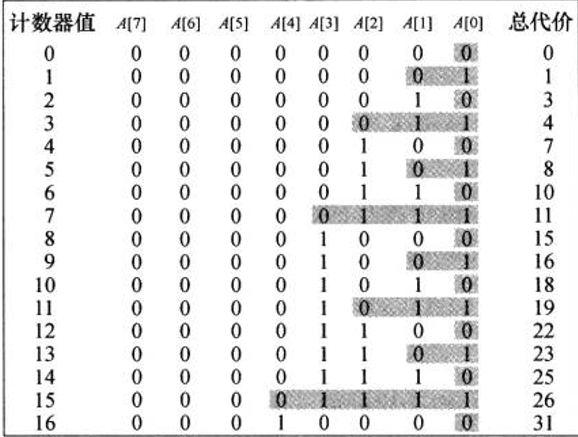聚合分析：二进制计数器

• 时间:

## 代码 ##
#include <iostream>
using namespace std;
void count(int a[], int n)
{
int i = 0;
while(i < n && a[i] == 1)
{
a[i] = 0;
i++;
}
if(i < n)
{
a[i] = 1;
}
}
/*测试程序*/
int main(int argc, char const *argv[])
{
int array = {0, 0,0, 0, 0, 0};

for(int i = 0; i <= 21; i++)
{
count(array, 6);
}
for(int i = 5; i >= 0; i--)
{
cout << array[i] << " ";
}
return 0;
}
## 这个图是我看了一个下午才理解其中的含义 ##